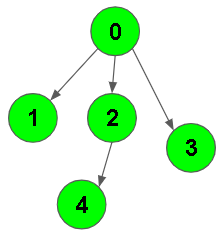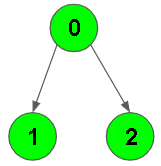XDAYS

:

HOUR

:

MINS

:

SEC

Copied to Clipboard
BFS of graph
Easy Accuracy: 42.71% Submissions: 100k+ Points: 2

Given a directed graph. The task is to do Breadth First Traversal of this graph starting from 0.
Note: One can move from node u to node v only if there's an edge from u to v and find the BFS traversal of the graph starting from the 0th vertex,
from left to right according to the graph. Also, you should only take nodes directly or indirectly connected from Node 0 in consideration.

Example 1:

Input:Output: 0 1 2 3 4
Explanation:
0 is connected to 1 , 2 , 3.
2 is connected to 4.
so starting from 0, it will go to 1 then 2
then 3.After this 2 to 4, thus bfs will be
0 1 2 3 4.


Example 2:

Input:Output: 0 1 2
Explanation:
0 is connected to 1 , 2.
so starting from 0, it will go to 1 then 2,
thus bfs will be 0 1 2 3 4.


You don’t need to read input or print anything. Your task is to complete the function bfsOfGraph() which takes the integer V denoting the number of vertices and adjacency list as input parameters and returns
a list containing the BFS traversal of the graph starting from the 0th vertex from left to right.

Expected Time Complexity: O(V + E)
Expected Auxiliary Space: O(V)

Constraints:
1 ≤ V, E ≤ 104

### Editorial

We strongly recommend solving this problem on your own before viewing its editorial. Do you still want to view the editorial?

#### My Submissions: